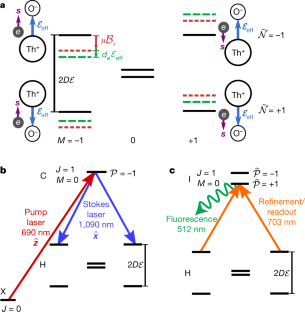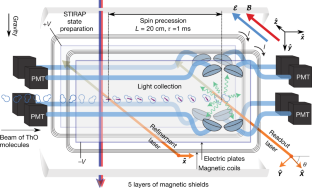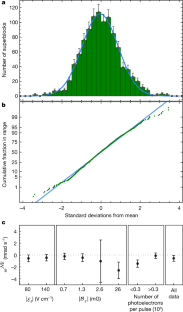Thank you for visiting nature.com. You are using a browser version with limited support for CSS. To obtain the best experience, we recommend you use a more up to date browser (or turn off compatibility mode in Internet Explorer). In the meantime, to ensure continued support, we are displaying the site without styles and JavaScript.

# Improved limit on the electric dipole moment of the electron

## Abstract

The standard model of particle physics accurately describes all particle physics measurements made so far in the laboratory. However, it is unable to answer many questions that arise from cosmological observations, such as the nature of dark matter and why matter dominates over antimatter throughout the Universe. Theories that contain particles and interactions beyond the standard model, such as models that incorporate supersymmetry, may explain these phenomena. Such particles appear in the vacuum and interact with common particles to modify their properties. For example, the existence of very massive particles whose interactions violate time-reversal symmetry, which could explain the cosmological matter–antimatter asymmetry, can give rise to an electric dipole moment along the spin axis of the electron. No electric dipole moments of fundamental particles have been observed. However, dipole moments only slightly smaller than the current experimental bounds have been predicted to arise from particles more massive than any known to exist. Here we present an improved experimental limit on the electric dipole moment of the electron, obtained by measuring the electron spin precession in a superposition of quantum states of electrons subjected to a huge intramolecular electric field. The sensitivity of our measurement is more than one order of magnitude better than any previous measurement. This result implies that a broad class of conjectured particles, if they exist and time-reversal symmetry is maximally violated, have masses that greatly exceed what can be measured directly at the Large Hadron Collider.

This is a preview of subscription content, access via your institution

## Relevant articles

• ### New benchmark scenarios of electroweak baryogenesis in aligned two Higgs double models

Journal of High Energy Physics Open Access 15 September 2022

• ### Complementary signals of lepton flavor violation at a high-energy muon collider

Journal of High Energy Physics Open Access 06 July 2022

• ### CP-violating axion interactions in effective field theory

Journal of High Energy Physics Open Access 04 July 2022

## Access options

\$32.00

All prices are NET prices.Fig. 1: Energy levels of thorium monoxide and laser transitions.Fig. 2: Schematic of the measurement region.Fig. 3: Statistics of the EDM dataset.

## Data availability

The data that support the conclusions of this article are available from the corresponding authors on reasonable request.

## References

1. Baron, J. et al. Order of magnitude smaller limit on the electric dipole moment of the electron. Science 343, 269–272 (2014).

2. Pospelov, M. E. & Khriplovich, I. B. Electric dipole moment of the W boson and the electron in the Kobayashi–Maskawa model. Sov. J. Nucl. Phys. 53, 638–640 (1991).

3. Pospelov, M. & Ritz, A. CKM benchmarks for electron electric dipole moment experiments. Phys. Rev. D 89, 056006 (2014).

4. Nakai, Y. & Reece, M. Electric dipole moments in natural super symmetry. J. High Energy Phys. 8, 31 (2017).

5. Barr, S. M. A review of CP violation in atoms. Int. J. Mod. Phys. A 08, 209–236 (1993).

6. Pospelov, M. & Ritz, A. Electric dipole moments as probes of new physics. Ann. Phys. 318, 119–169 (2005).

7. Engel, J., Ramsey-Musolf, M. J. & van Kolck, U. Electric dipole moments of nucleons, nuclei, and atoms: the standard model and beyond. Prog. Part. Nucl. Phys. 71, 21–74 (2013).

8. Bernreuther, W. & Suzuki, M. The electric dipole moment of the electron. Rev. Mod. Phys. 63, 313–340 (1991).

9. ACME Collaboration et al. Methods, analysis, and the treatment of systematic errors for the electron electric dipole moment search in thorium monoxide. New J. Phys. 19, 073029 (2016).

10. Hudson, J. J. et al. Improved measurement of the shape of the electron. Nature 473, 493–496 (2011).

11. Kara, D. M. et al. Measurement of the electron’s electric dipole moment using YbF molecules: methods and data analysis. New J. Phys. 14, 103051 (2012).

12. Cairncross, W. B. et al. Precision measurement of the electron’s electric dipole moment using trapped molecular ions. Phys. Rev. Lett. 119, 153001 (2017).

13. Sandars, P. G. H. The electric dipole moment of an atom. Phys. Lett. 14, 194–196 (1965).

14. Khriplovich, I. B. & Lamoreaux, S. K. CP Violation Without Strangeness (Springer, NewYork, 1997).

15. Commins, E. D. & DeMille, D. in Lepton Dipole Moments (eds Roberts, B. L. & Marciano, W. J.) Ch. 14 (World Scientific, Singapore, 2010).

16. Denis, M. & Fleig, T. In search of discrete symmetry violations beyond the standard model: thorium monoxide reloaded. J. Chem. Phys. 145, 214307 (2016).

17. Skripnikov, L. V. Combined 4-component and relativistic pseudo potential study of ThO for the electron electric dipole moment search. J. Chem. Phys. 145, 214301 (2016).

18. Vutha, A. C. et al. Search for the electric dipole moment of the electron with thorium monoxide. J. Phys. B 43, 074007 (2010).

19. Regan, B. C., Commins, E. D., Schmidt, C. J. & DeMille, D. New limit on the electron electric dipole moment. Phys. Rev. Lett. 88, 071805 (2002).

20. Bickman, S., Hamilton, P., Jiang, Y. & DeMille, D. Preparation and detection of states with simultaneous spin alignment and selectable molecular orientation in PbO. Phys. Rev. A 80, 023418 (2009).

21. Eckel, S., Hamilton, P., Kirilov, E., Smith, H. W. & DeMille, D. Search for the electron electric dipole moment using -doublet levels in PbO. Phys. Rev. A 87, 052130 (2013).

22. Kirilov, E. et al. Shot-noise-limited spin measurements in a pulsed molecular beam. Phys. Rev. A 88, 013844 (2013).

23. Hutzler, N. R., Lu, H. I. & Doyle, J. M. The buffer gas beam: an intense, cold, and slow source for atoms and molecules. Chem. Rev. 112, 4803–4827 (2012).

24. Hutzler, N. R. et al. A cryogenic beam of refractory, chemically reactive molecules with expansion cooling. Phys. Chem. Chem. Phys. 13, 18976 (2011).

25. Patterson, D. & Doyle, J. M. Bright, guided molecular beam with hydrodynamic enhancement. J. Chem. Phys. 126, 154307 (2007).

26. Panda, C. D. et al. Stimulated Raman adiabatic passage preparation of a coherent superposition of ThO H3Δ1 states for an improved electron electric-dipole-moment measurement. Phys. Rev. A 93, 052110 (2016).

27. Gray, H. R., Whitley, R. M. & Stroud, C. R. Coherent trapping of atomic populations. Opt. Lett. 3, 218–220 (1978).

28. Kokkin, D. L., Steimle, T. C. & DeMille, D. Branching ratios and radiative lifetimes of the U, L, and i states of thorium oxide. Phys. Rev. A 90, 062503 (2014).

29. Kokkin, D. L., Steimle, T. C. & DeMille, D. Characterization of the I(| = 1) − X 1Σ+ (0, 0) band of thorium oxide. Phys. Rev. A 91, 042508 (2015).

30. Huber, P. J. Robust estimation of a location parameter. Ann. Math. Stat. 35, 73–101 (1964).

31. Efron, B. Bootstrap methods: another look at the jackknife. Ann. Stat. 7, 1–26 (1979).

32. Efron, B. & Tibshirani, R. Bootstrap Methods for standard errors, confidence intervals, and other measures of statistical accuracy. Stat. Sci. 1, 54–75 (1986).

33. Feldman, G. J. & Cousins, R. D. Unified approach to the classical statistical analysis of small signals. Phys. Rev. D 57, 3873–3889 (1998).

34. Kozlov, M. G. & Labzowsky, L. N. Parity violation effects in diatomic molecules. J. Phys. B 28, 1933–1961 (1995).

35. Dzuba, V. A., Flambaum, V. V. & Harabati, C. Relations between matrix elements of different weak interactions and interpretation of the parity-nonconserving and electron electric-dipole-moment measurements in atoms and molecules. Phys. Rev. A 84, 052108 (2011).

36. Fortson, N., Sandars, P. & Barr, S. The search for a permanent electric dipole moment. Phys. Today 56, 33–39 (2003).

37. Andreev, V., Panda, C. D., Hess, P. W., Spaun, B. & Gabrielse, G. A self-calibrating polarimeter to measure Stokes parameters. Preprint at https://arxiv.org/abs/1703.00963 (2017).

38. Kenney, J. F. & Keeping, E. S. Mathematics of Statistics: Part One 4th edn (Chapman & Hall, London, 1954).

39. Shuman, E. S., Barry, J. F., Glenn, D. R. & DeMille, D. Radiative force from optical cycling on a diatomic molecule. Phys. Rev. Lett. 103, 223001 (2009).

## Acknowledgements

This work was supported by the NSF. J.H. was supported by the Department of Defense. D.G.A. was partially supported by the Amherst College Kellogg University Fellowship. We thank M. Reece and M. Schwartz for discussions and S. Cotreau, J. MacArthur and S. Sansone for technical support.

### Reviewer information

Nature thanks E. Hinds and Y. Shagam for their contribution to the peer review of this work.

## Author information

### Contributions

All authors contributed to one or more of the following areas: proposing, leading and running the experiment; design, construction, optimization and testing of the experimental apparatus and data acquisition system; setup and maintenance during the data runs; data analysis and extraction of physics results from measured traces; modelling and simulation of systematic errors; and the writing of this article. The corresponding authors are D.D., J.M.D. and G.G. (acme@physics.harvard.edu).

### Corresponding author

Correspondence to D. DeMille.

## Ethics declarations

### Competing interests

The authors declare no competing interests.

Publisher’s note: Springer Nature remains neutral with regard to jurisdictional claims in published maps and institutional affiliations.

## Extended data figures and tables

### Extended Data Fig. 1 Switching timescales.

a, Fluorescence signal amplitude versus time in an $$\hat{{\boldsymbol{X}}},\hat{{\boldsymbol{Y}}}$$ polarization cycle. The red line corresponds to the signal from the $$\hat{{\boldsymbol{X}}}$$-polarization laser and the black line to the signal from the $$\hat{{\boldsymbol{Y}}}$$-polarization laser. b, Measured molecular trace (25 averaged pulses) versus time. Signal averaged over the entire $$\hat{{\boldsymbol{X}}},\hat{{\boldsymbol{Y}}}$$ polarization cycles shown in a are shown in red and black for the $$\hat{{\boldsymbol{X}}}$$ and $$\hat{{\boldsymbol{Y}}}$$ laser polarizations, respectively. c, Switches performed within a block. The $$\tilde{\mathcal{N}}$$ and $$\tilde{\mathcal{B}}$$ switches randomly alternate between a (−+) and a (+−) pattern, and the $$\tilde{\mathcal{E}}$$ and $$\tilde{\theta}$$ switches randomly alternate between (−++−) and (+−−+) between blocks. d, Switches performed within a superblock. The $$\tilde{\mathcal{P}}$$-state order is selected randomly, while $$\tilde{\mathcal{L}}$$ and $$\tilde{\mathcal{R}}$$ are deterministic. e, Run-data structure. We alternate between ‘normal’ EDM data, taken at three values of $$|{{\mathcal{B}}}_{z}|$$, and monitoring of known systematic effects by performing intentional parameter variations (IPVs). For several days data were taken with $$|{{\mathcal{B}}}_{z}|=2.6\,{\rm{m}}{\rm{G}}$$ instead of $$|{{\mathcal{B}}}_{z}|=0.7\,{\rm{m}}{\rm{G}}$$, which is shown in the figure. Each IPV corresponds to one superblock, where a control parameter (AE) is deliberately offset from its ideal value. Here, A = Pref (the refinement beam is completely blocked, to determine the intrinsic $${\omega }_{{\rm{S}}{\rm{T}}}^{{\mathcal{N}}{\mathcal{E}}}$$), $$B={{\mathcal{E}}}^{{\rm{n}}{\rm{r}}}$$, $$C={P}^{{\mathcal{N}}{\mathcal{E}}}$$, $$D={\phi }_{{\rm{S}}{\rm{T}}}^{{\mathcal{N}}{\mathcal{E}}}$$ and $$E={\rm{\partial }}{{\mathcal{B}}}_{z}/{\rm{\partial }}z$$. The magnetic-field magnitude for the IPV of parameter E was varied between three experimental values within a run. f, The EDM dataset. The electric-field magnitude was varied from day to day. The magnetic-field magnitude for the IPVs for parameters A, B, C and D was varied between three experimental values.

### Extended Data Fig. 2 The $${\boldsymbol{\partial }}{\pmb{\mathcal{B}}}_{{\boldsymbol{z}}}/{\boldsymbol{\partial }}{\boldsymbol{z}}\times {\boldsymbol{\delta }}\times {\boldsymbol{\partial }}{\pmb{\mathcal{E}}}^{{\bf{n}}{\bf{r}}}/{\boldsymbol{\partial }}{\boldsymbol{z}}$$ systematic error.

a, A $${\rm{\partial }}{{\mathcal{E}}}^{{\rm{n}}{\rm{r}}}/{\rm{\partial }}z$$ gradient (blue arrows) causes a z-dependent two-photon detuning correlated with $${\mathcal{N}}{\mathcal{E}}$$ ($${\delta }_{z}^{{\mathcal{N}}{\mathcal{E}}}$$), due to the Stark shift $$D{\mathcal{E}}$$. When δ ≠ 0, the combination of a non-zero $${\delta }_{z}^{{\mathcal{N}}{\mathcal{E}}}$$ and a dependence of the STIRAP efficiency on the two-photon detuning, ∂η/∂δ (shown as black lines), acts to translate the detected molecular cloud (purple gradient ellipse) position by $${\rm{d}}{z}_{{\rm{c}}{\rm{m}}}^{{\mathcal{N}}{\mathcal{E}}}$$ (purple arrow). A non-zero $${\rm{\partial }}{{\mathcal{B}}}_{z}/{\rm{\partial }}z$$ (teal-colour gradient) causes molecules to accumulate more (less) precession phase if their position has a smaller (larger) z coordinate. The effects combine to create the dependence of $${\omega }^{{\mathcal{N}}{\mathcal{E}}}$$ on $${\rm{\partial }}{{\mathcal{B}}}_{z}/{\rm{\partial }}z$$. The scales are exaggerated for clarity. b, The effect of changing the STIRAP two-photon detuning, δ, on the $${\omega }^{{\mathcal{N}}{\mathcal{E}}}$$ versus $${\rm{\partial }}{{\mathcal{B}}}_{z}/{\rm{\partial }}z$$. We note that the slope $${\rm{\partial }}{\omega }^{{\mathcal{N}}{\mathcal{E}}}/{\rm{\partial }}({\rm{\partial }}{{\mathcal{B}}}_{z}/{\rm{\partial }}z)$$ is consistent with zero when δ is set to zero. c, Dependence of $${\omega }^{{\mathcal{N}}{\mathcal{E}}}$$ on δ and $${\rm{\partial }}{{\mathcal{B}}}_{z}/{\rm{\partial }}z$$. Fits (dashed curves) to a simple lineshape model (see Methods) show good agreement with the data. δ = 0 is defined as the point where all curves cross. The error bars in b and c represent 1σ statistical uncertainties.

## Supplementary information

### Supplementary Information

The supplementary methods section contains text describing in detail the mechanisms leading to the systematics effects referenced in the main text.

## Rights and permissions

Reprints and Permissions

• ### Searching for BSM physics in Yukawa couplings and flavour symmetries

• J. Alonso-González
• A. de Giorgi
• S. Pokorski

Journal of High Energy Physics (2022)

• ### Electroweak baryogenesis in aligned two Higgs doublet models

• Kazuki Enomoto
• Shinya Kanemura
• Yushi Mura

Journal of High Energy Physics (2022)

• ### A theory of dark pions

• Hsin-Chia Cheng
• Lingfeng Li
• Ennio Salvioni

Journal of High Energy Physics (2022)

• ### CP-violating 2HDMs emerging from 3-3-1 models

• Zhiyi Fan
• Kei Yagyu

Journal of High Energy Physics (2022)

• ### Pseudo-Goldstone dark matter model with CP violation

• Neda Darvishi

Journal of High Energy Physics (2022)